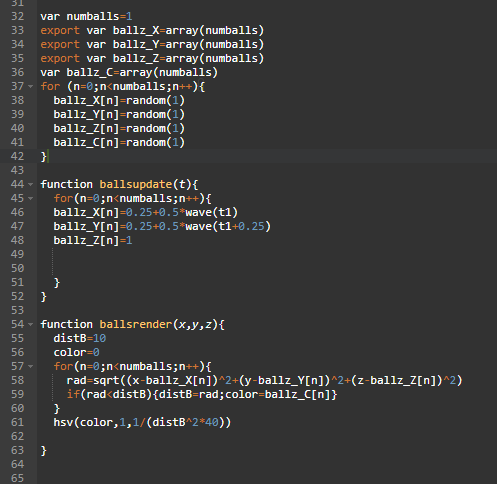# Square root returns negative values

I have a function that tries to move a simulated spot around a 3D volume. One of the things I calculate along the way is the radius to the nearest spot. I’m using the standard Pythagorean distance ie r=((X-x)^2+(Y-y)^2+(Z-z)^2)^0.5. I’m watching the output of r on the vars watch panel, and I see that sometimes it just comes out as a negative value. I have the X,Y,and Z position of the object moving continuously, and the output absolute value of r is relatively continuous, but occasionally it appears to just change signs. It does this regardless of using ()^0.5 or sqrt(). Usually the output of a square root should be taken as positive if the input is positive? Do I have an error here?Caret ‘^’ is a bitwise xor operator. You’ll want ‘sqrt()’ for sure. And for raising to the power of 2, it’s best to put the value to square in a var and multiply it by itself `x*x`. You can also use `pow(x,2)` but it’s slower.

If your values are over 32767, you can overflow to negative.

1 Like

That seems to fix it.

1 Like